NEET  >  31 Year NEET Previous Year Questions: Thermodynamics - 2

# 31 Year NEET Previous Year Questions: Thermodynamics - 2

Test Description

## 30 Questions MCQ Test Physics Class 11 | 31 Year NEET Previous Year Questions: Thermodynamics - 2

31 Year NEET Previous Year Questions: Thermodynamics - 2 for NEET 2023 is part of Physics Class 11 preparation. The 31 Year NEET Previous Year Questions: Thermodynamics - 2 questions and answers have been prepared according to the NEET exam syllabus.The 31 Year NEET Previous Year Questions: Thermodynamics - 2 MCQs are made for NEET 2023 Exam. Find important definitions, questions, notes, meanings, examples, exercises, MCQs and online tests for 31 Year NEET Previous Year Questions: Thermodynamics - 2 below.
Solutions of 31 Year NEET Previous Year Questions: Thermodynamics - 2 questions in English are available as part of our Physics Class 11 for NEET & 31 Year NEET Previous Year Questions: Thermodynamics - 2 solutions in Hindi for Physics Class 11 course. Download more important topics, notes, lectures and mock test series for NEET Exam by signing up for free. Attempt 31 Year NEET Previous Year Questions: Thermodynamics - 2 | 30 questions in 60 minutes | Mock test for NEET preparation | Free important questions MCQ to study Physics Class 11 for NEET Exam | Download free PDF with solutions
 1 Crore+ students have signed up on EduRev. Have you?
31 Year NEET Previous Year Questions: Thermodynamics - 2 - Question 1

### First law of thermodynamics is consequence of conservation of 

Detailed Solution for 31 Year NEET Previous Year Questions: Thermodynamics - 2 - Question 1

The first law of thermodynamics is just a conservation of energy.

31 Year NEET Previous Year Questions: Thermodynamics - 2 - Question 2

### At 27° Ca gas is compressed suddenly such that its pressure becomes (1/8) of original pressure. Final temperature will be (γ = 5/3)

Detailed Solution for 31 Year NEET Previous Year Questions: Thermodynamics - 2 - Question 2

Temperature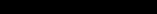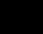Pressure becomes (1/8)th of its original pressure.

We know that,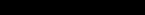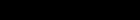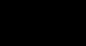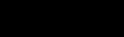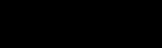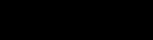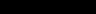31 Year NEET Previous Year Questions: Thermodynamics - 2 - Question 3

### A thermodynamic process is shown in the figure. The pressures and volumes corresponding to some points in the figure are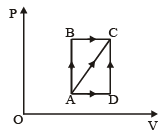PA = 3 × 104 Pa VA = 2 × 10-3 m3 PB = 8 × 104 Pa VD = 5 × 10–3 m3. In process AB, 600 J of heat is added to the system and in process BC, 200 J of heat is added to the system. The change in internal energy of the system in process AC would be 

Detailed Solution for 31 Year NEET Previous Year Questions: Thermodynamics - 2 - Question 3

Since AB is an isochoric process, so, no work is done. BC is isobaric process,
∴ W = PB × (VD – VA) = 240 J
ΔQ = 600 + 200 = 800 J
Using ΔQ = ΔU + ΔW
⇒ ΔU = ΔQ – ΔW = 800 – 240 = 560 J

31 Year NEET Previous Year Questions: Thermodynamics - 2 - Question 4

For hydrogen gas, Cp – Cv = a and for oxygen gas, Cp – Cv = b, so the relation between a and b is given by 

Detailed Solution for 31 Year NEET Previous Year Questions: Thermodynamics - 2 - Question 4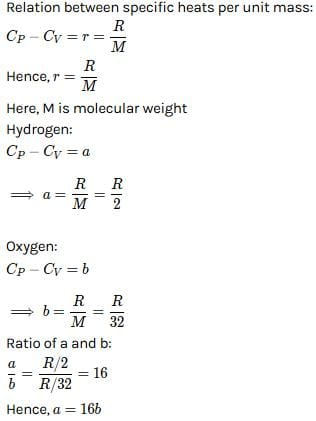31 Year NEET Previous Year Questions: Thermodynamics - 2 - Question 5

If for a gas,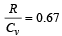, the gas is made up of molecules which are 

Detailed Solution for 31 Year NEET Previous Year Questions: Thermodynamics - 2 - Question 5

Since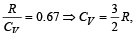hence gas is monoatomic.

31 Year NEET Previous Year Questions: Thermodynamics - 2 - Question 6

A thermodynamic system is taken from state A to B along ACB and is brought back to A along BDA  as shown in the PV diagram. The net work done during the complete cycle is given by the area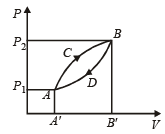Detailed Solution for 31 Year NEET Previous Year Questions: Thermodynamics - 2 - Question 6

Work done = Area under curve ACBDA

31 Year NEET Previous Year Questions: Thermodynamics - 2 - Question 7

An ideal gas A and a real gas B have their volumes increased from V to 2V under isothermal conditions. The increase in internal energy 

Detailed Solution for 31 Year NEET Previous Year Questions: Thermodynamics - 2 - Question 7

Under isothermal conditions, there is no change in internal energy.

31 Year NEET Previous Year Questions: Thermodynamics - 2 - Question 8

110 joules of heat is added to a gaseous system, whose internal energy is 40J; then the amount of external work done is 

Detailed Solution for 31 Year NEET Previous Year Questions: Thermodynamics - 2 - Question 8

ΔQ = ΔU + ΔW
⇒   ΔW = ΔQ  – ΔU = 110 – 40 = 70 J

31 Year NEET Previous Year Questions: Thermodynamics - 2 - Question 9

Which of the following is not thermodynamical function ? 

Detailed Solution for 31 Year NEET Previous Year Questions: Thermodynamics - 2 - Question 9

Work done is not a thermodynamical function.

31 Year NEET Previous Year Questions: Thermodynamics - 2 - Question 10

An ideal carnot engine, whose efficiency is 40% receives heat at 500 K. If its efficiency is 50%, then the intake temperature for the same exhaust temperature is 

Detailed Solution for 31 Year NEET Previous Year Questions: Thermodynamics - 2 - Question 10

Efficiency of carnot engine (η1) = 40% = 0.4; Initial intake temperature (T1) = 500K and new efficiency (η2) = 50% = 0.5.
Efficiency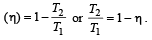Therefore in first case,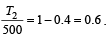⇒  T2 = 0.6×500=300K

And in second case,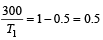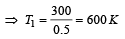31 Year NEET Previous Year Questions: Thermodynamics - 2 - Question 11

An ideal gas undergoing adiabatic change has the following pressure-temperature relationship 

Detailed Solution for 31 Year NEET Previous Year Questions: Thermodynamics - 2 - Question 11

We know that in adiabatic process, PVγ = constant ....(1)
From ideal gas equation, we know that PV = nRT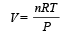....(2)
Puttingt the value from equation  (2) in equation (1),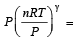constant

P(1 – γ) Tγ = constant

31 Year NEET Previous Year Questions: Thermodynamics - 2 - Question 12

A diatomic gas initially at 18ºC is compressed adiabatically to one eighth of its original volume.The temperature after compression will be 

Detailed Solution for 31 Year NEET Previous Year Questions: Thermodynamics - 2 - Question 12

Initial temperature (T1) = 18°C = 291 K
Let Initial volume (V1) = V

Final volume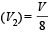According to adiabatic process, TVγ – 1 = constant According to question,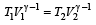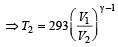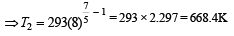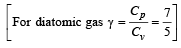31 Year NEET Previous Year Questions: Thermodynamics - 2 - Question 13

A sample of gas expands from volume V1 to V2.The amount of work done by the gas is greatest, when the expansion is 

Detailed Solution for 31 Year NEET Previous Year Questions: Thermodynamics - 2 - Question 13

In thermodynamics for same change in volume, the work done is maximum in isobaric process because in P – V graph, area enclosed by curve and volume axis is maximum in isobaric process.
So, the choice (b) is correct.

31 Year NEET Previous Year Questions: Thermodynamics - 2 - Question 14

The efficiency of a Carn ot engine operating between the temperatures of 100ºC and –23ºC will be


Detailed Solution for 31 Year NEET Previous Year Questions: Thermodynamics - 2 - Question 14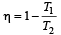T1 = –23°C = 250 K,   T2 = 100°C = 373K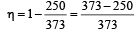31 Year NEET Previous Year Questions: Thermodynamics - 2 - Question 15

If the ratio of specific heat of a gas at constant pressure to that at constant volume is γ, the change in internal energy of a mass of gas, when the volume changes from V to 2V at constant pressure P, is 

Detailed Solution for 31 Year NEET Previous Year Questions: Thermodynamics - 2 - Question 15

Change in internal energy is equal to work done in adiabatic system ΔW = –ΔU   (Expansion in the system)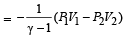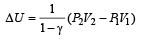Here,  V1 = V ,V2 = 2V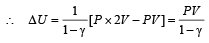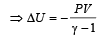31 Year NEET Previous Year Questions: Thermodynamics - 2 - Question 16

We consider a thermodynamic system. If ΔU represents the increase in its internal energy and W the work done by the system, which of the following statements is true? 

Detailed Solution for 31 Year NEET Previous Year Questions: Thermodynamics - 2 - Question 16

ΔQ = ΔU + W
For adiabatic process, ΔQ = 0
ΔU = –W

31 Year NEET Previous Year Questions: Thermodynamics - 2 - Question 17

An ideal gas at 27ºC is compressed adiabatically to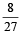of its original volume. The rise in temperature is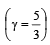Detailed Solution for 31 Year NEET Previous Year Questions: Thermodynamics - 2 - Question 17

(T2/T1) = (V2/V1)γ-1
(T2/300) = (23/33)2/3
T2 = 675K
∆T = 375K

31 Year NEET Previous Year Questions: Thermodynamics - 2 - Question 18

A reversible engine converts one-sixth of the heat input into work. When the temperature of the sink is reduced by 62ºC, the efficiency of the engine is doubled. The temperatures of the source and sink are 

Detailed Solution for 31 Year NEET Previous Year Questions: Thermodynamics - 2 - Question 18

Initially the efficiency of the engine was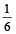which increases to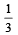when the sinktemperature reduces by 62º C.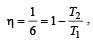when T2 = sink temperature T1 = source temperature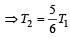Secondly,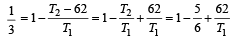or, T1 = 62 × 6 = 372K =372– 273 = 99ºC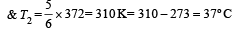31 Year NEET Previous Year Questions: Thermodynamics - 2 - Question 19

If γ be the ratio of specific heats of a perfect gas, the number of degrees of freedom of a molecule of the gas is 

Detailed Solution for 31 Year NEET Previous Year Questions: Thermodynamics - 2 - Question 19

We know that ratio of specific heats,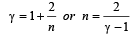[where n = Degree of freedom]

31 Year NEET Previous Year Questions: Thermodynamics - 2 - Question 20

The temperature of source and sink of a heat engine are 127ºC and 27ºC respectively. An inventor claims its efficiency to be 26%, then:

Detailed Solution for 31 Year NEET Previous Year Questions: Thermodynamics - 2 - Question 20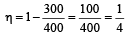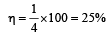Hence, it is not possible to have efficiency more than 25%.

31 Year NEET Previous Year Questions: Thermodynamics - 2 - Question 21

A gas at 27ºC temperature and 30 atmospheric pressure is allowed to expand to the atmospheric pressure. If the volume becomes 10 times its initial volume, then the final temperature becomes

Detailed Solution for 31 Year NEET Previous Year Questions: Thermodynamics - 2 - Question 21

Given :  Initial temperature of gas (T1) = 27°C = 300 K
Initial pressure (P1) = 30 atm Initial volume (V1) = V
Final pressure (P2) = 1 atm Final volume (V2) = 10 V.
We know from the general gas  equation that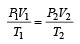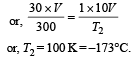31 Year NEET Previous Year Questions: Thermodynamics - 2 - Question 22

A Carnot engine whose efficiency is 50% has an exhaust temperature of 500 K. If the efficiency is to be 60% with the same intake temperature, the exhaust temperature must be (in K) 

Detailed Solution for 31 Year NEET Previous Year Questions: Thermodynamics - 2 - Question 22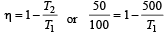⇒  T1 = 1000K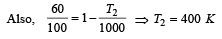31 Year NEET Previous Year Questions: Thermodynamics - 2 - Question 23

An ideal gas heat engine operates in a Carnot cycle between 227ºC and 127ºC. It absorbs 6 kcal at the higher temperature. The amount of heat (in kcal) converted into work is equal to

Detailed Solution for 31 Year NEET Previous Year Questions: Thermodynamics - 2 - Question 23

Efficiency =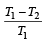T1 = 227 + 273 = 500 K
T2 = 127 + 273 = 400 K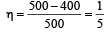Hence,  output work

= (η) Heat input =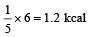31 Year NEET Previous Year Questions: Thermodynamics - 2 - Question 24

One mole of an ideal gas at an initial temperature of T K does 6R joules of work adiabatically. If the ratio of specific heats of this gas at constant pressure and at constant volume is 5/3, the final temperature of gas will be 

Detailed Solution for 31 Year NEET Previous Year Questions: Thermodynamics - 2 - Question 24

T1 = T, W = 6R joules,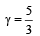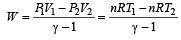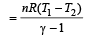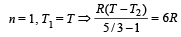⇒  T2 = ( T–4)K

31 Year NEET Previous Year Questions: Thermodynamics - 2 - Question 25

Which of the following processes is reversible?

Detailed Solution for 31 Year NEET Previous Year Questions: Thermodynamics - 2 - Question 25

For a process to be reversible, it must be quasi-static. For quasi static process, all changes take place infinitely slowly.
Isothermal process occur very slowly so it is quasi-static and hence it is reversible.

31 Year NEET Previous Year Questions: Thermodynamics - 2 - Question 26

An ideal gas heat engine oper ates in Car not cycle between 227°C and 127°C. It absorbs 6 × 104 cals of heat at higher temperature. Amount of heat converted to work is 

Detailed Solution for 31 Year NEET Previous Year Questions: Thermodynamics - 2 - Question 26

We know that efficiency of carnot engine =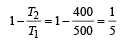[∵ T1 = (273 + 227)K = 500 K
and T2 = (273 + 127)K = 400 K]

Efficiency of Heat engine  =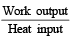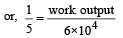⇒ work output = 1.2 × 104 cal

31 Year NEET Previous Year Questions: Thermodynamics - 2 - Question 27

A Carnot engine whose sink is at 300 K has an efficiency of 40%. By how much should the temperature of source be increased so as to increase, its efficiency by 50% of original efficiency ? 

Detailed Solution for 31 Year NEET Previous Year Questions: Thermodynamics - 2 - Question 27

We know that efficiency of Carnot Engine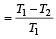where, T1 is temp. of source & T2 is temp. of sink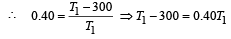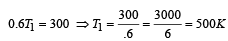Now efficiency to be increased by 50%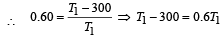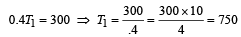Increase in temp = 750 – 500 = 250 K

31 Year NEET Previous Year Questions: Thermodynamics - 2 - Question 28

The molar specific heat at constant pressure of an ideal gas is (7/2) R. The ratio of specific heat at constant pressure to that at constant volume is

Detailed Solution for 31 Year NEET Previous Year Questions: Thermodynamics - 2 - Question 28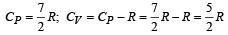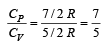31 Year NEET Previous Year Questions: Thermodynamics - 2 - Question 29

An engine has an efficiency of 1/6. When the temperature of sink is reduced by 62°C, its efficiency is doubled. Temperature of the source is

Detailed Solution for 31 Year NEET Previous Year Questions: Thermodynamics - 2 - Question 29

Since efficiency of engine is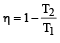According to problem,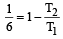.......... (1)

When the temperature of the sink is reduced by 62°C, its efficiency is doubled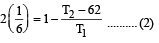Solving (1) and (2) T2 = 372K
T1 = 99°C = Temperature of source.

31 Year NEET Previous Year Questions: Thermodynamics - 2 - Question 30

If Q, E and W denote respectively the heat added, change in internal energy and the work done in a closed cyclic process, then: 

Detailed Solution for 31 Year NEET Previous Year Questions: Thermodynamics - 2 - Question 30

In a cyclic process, the initial state coincides with the final state. Hence, the change in internal energy is zero, as it depends only on the initial and final states. But Q & W are non-zero during a cycle process.

## Physics Class 11

127 videos|464 docs|210 tests
 Use Code STAYHOME200 and get INR 200 additional OFF Use Coupon Code
Information about 31 Year NEET Previous Year Questions: Thermodynamics - 2 Page
In this test you can find the Exam questions for 31 Year NEET Previous Year Questions: Thermodynamics - 2 solved & explained in the simplest way possible. Besides giving Questions and answers for 31 Year NEET Previous Year Questions: Thermodynamics - 2, EduRev gives you an ample number of Online tests for practice

## Physics Class 11

127 videos|464 docs|210 tests

### How to Prepare for NEET

Read our guide to prepare for NEET which is created by Toppers & the best Teachers